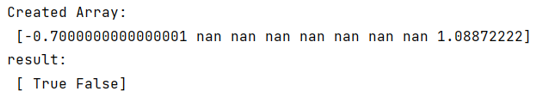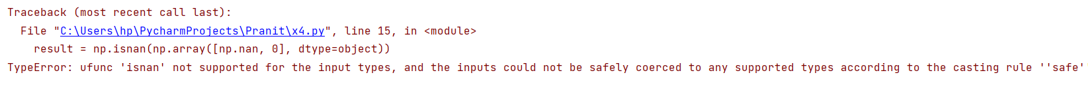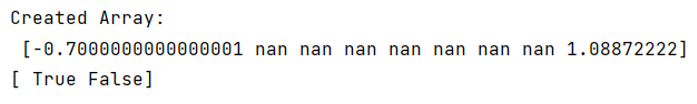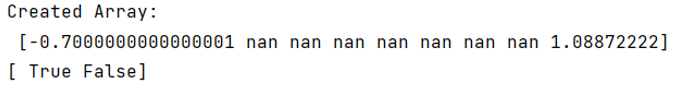# NumPy isnan() fails on an array of floats

Learn why NumPy isnan() fails on an array of floats, and what we should use instead of this? By Pranit Sharma Last updated : September 23, 2023

NumPy is an abbreviated form of Numerical Python. It is used for different types of scientific operations in Python. Numpy is a vast library in Python which is used for almost every kind of scientific or mathematical operation. It is itself an array that is a collection of various methods and functions for processing the arrays.

## numpy.isnan() fails on an array of floats

The numpy.isnan() usually fails on values having data type float, or it fails when it comes to data type object. We should rather use numpy.isnull() instead of isnan() to make the process more effective. It can accept NumPy arrays of an object or native dtypes.

Note

To work with pandas, we need to import pandas package first, below is the syntax:

```import pandas as pd
```

Let us understand with the help of an example,

## Python program to demonstrate example of NumPy isnan() fails on an array of floats

```# Importing numpy package
import numpy as np

# Creating an array
arr = np.array([-0.7000000000000001, np.NaN,np.NaN,np.NaN,np.NaN,np.NaN,np.NaN,np.NaN,1.08872222000],dtype=object)

# Print array
print("Created Array:\n",arr)

# Applying isnan
result = np.isnan(np.array([np.nan, 0], dtype=np.float64))

print("result:\n",result)
```

Output:## Using dtype=object

This code fails when we put dtype=object.

```# Applying isnan
result = np.isnan(np.array([np.nan, 0], dtype=object))

print("result:\n",result)
```

Output:## Fix this error by using isnull()

To overcome this, we will use isnull().

```# Applying isnaull
print(pd.isnull(np.array([np.nan, 0], dtype=float)))
```

Output:```print(pd.isnull(np.array([np.nan, 0], dtype=object)))
```

Output: